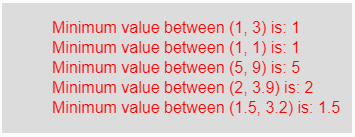# p5.js | min() function

The min() function in p5.js is used to get the minimum value from the sequence of numbers.

Syntax:

`min(a, b)`

or

`min(arr)`

Parameters: The min(a, b) function accepts two parameters which are two different number and compared to get minimum value among them. The min(arr) function accepts a single parameter array.

Return Value: It returns the minimum value among the different numbers.

Below program illustrates the min() function in p5.js:

Example: This example uses min() function to get the minimum value.

 `function` `setup() {  ` `  `  `    ``// Create Canvas of size 270*80  ` `    ``createCanvas(350, 130);  ` `}  ` `  `  `function` `draw() {  ` `      `  `    ``// Set the background color  ` `    ``background(220);  ` `      `  `    ``// Call to min() function  ` `    ``let u = min(1, 3); ` `    ``let v = min(1, 1); ` `    ``let w = min(5, 9); ` `    ``let x = min(2, 3.9); ` `    ``let y = min(1.5, 3.2); ` `      `  `    ``// Set the size of text  ` `    ``textSize(16);  ` `      `  `    ``// Set the text color  ` `    ``fill(color(``'red'``));  ` `    `  `    ``// Getting minimum value     ` `    ``text(``"Minimum value between (1, 3) is: "` `+ u, 50, 30); ` `    ``text(``"Minimum value between (1, 1) is: "` `+ v, 50, 50); ` `    ``text(``"Minimum value between (5, 9) is: "` `+ w, 50, 70); ` `    ``text(``"Minimum value between (2, 3.9) is: "` `+ x, 50, 90); ` `    ``text(``"Minimum value between (1.5, 3.2) is: "` `+ y, 50, 110);      ` `}  `

Output:Reference: https://p5js.org/reference/#/p5/minMy Personal Notes arrow_drop_upCheck out this Author's contributed articles.

If you like GeeksforGeeks and would like to contribute, you can also write an article using contribute.geeksforgeeks.org or mail your article to contribute@geeksforgeeks.org. See your article appearing on the GeeksforGeeks main page and help other Geeks.

Please Improve this article if you find anything incorrect by clicking on the "Improve Article" button below.

Article Tags :

Be the First to upvote.

Please write to us at contribute@geeksforgeeks.org to report any issue with the above content.